# An Overview of System-Level Macromodeling Using Virtual Blocks

## An Introduction to Macromodeling in RF.Spice A/D

RF.Spice A/D provides an extensive library of black-box virtual blocks that allow you to quickly test and verify new system concepts without getting into the details of particular circuit realizations. A virtual block typically has one or more input pins and one or more output pins. It models a system-level function or behavior in the form of a relationship between the input and output voltages. For example, a multiplier block takes two input voltages and outputs their product. An analog frequency doubler takes a single sinusoidal input voltage and produces a sinusoidal output voltage whose frequency is twice as large as the input frequency. A real practical frequency doubler typically involves a sophisticated circuit either with discrete components or in the form of an integrated circuit. In many situations like system design, you simply need a "black box" that exhibits the behavior of a frequency doubler just to try out and verify a system concept. You would worry about the hardware implementation of your block at a later time after the proof of concept has been established. RF.Spice's virtual blocks will prove very useful when you design complex systems that contain many subcircuits and subsystems.

One of the great strengths of RF.Spice A/D is the ease of creating new user-defined devices or models. Most of RF.Spice's virtual blocks are indeed based on preloaded parameterized subcircuit models. You can open the Netlist code of these subcircuits, copy or modify them and package them into your own new virtual blocks. You can even convert an entire circuit into a model complete with your own symbol. There are also a number of virtual blocks whose underpinning models have been hard-coded into RF.Spice's simulation engines and device manager. An important difference between black-box virtual blocks and user-defined devices or simulation models is that virtual blocks typically use idealized devices/models and a combination of linear and nonlinear sources to minimize circuit complexity regardless of the feasibility of their hardware realization. In other words, they are primarily intended to represent functional behaviors rather than practical circuits.

## The Stages of a Macromodel Virtual Block

A virtual block connects to the other parts of your circuit via its input and output pins. Most virtual blocks do not have a ground or reference pin. In that case, they are assumed to share the global ground. Almost all virtual blocks have input and output stages with either implicit or explicit input resistance and output resistance parameters. The default values of the input and output resistances are 10GΩ and 1μΩ, respectively. Obviously, these are unrealistic extreme values. The figure below shows the input and output stages of a typical virtual block. In cases where a virtual block does not provide explicit input and output resistance parameters, you can add an external shunt resistor at the input and an external series resistor at the output. The large shunt resistor at the output is added to provide better stability for DC operating point analysis.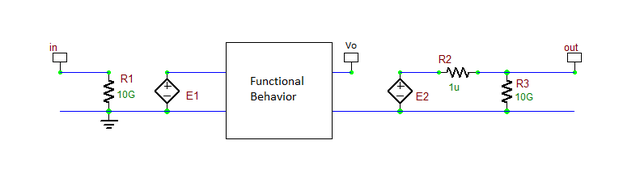A generic macromodel with input and output stages.

In the above figure, the linear controlled voltage source E1 usually follows the input voltage vin and serves as an input buffer. It might also be replaced with a linear controlled current source or with a nonlinear (B-type) controlled voltage or current source depending on the particular purpose of the macro model. Similarly, the linear controlled voltage source E2 usually follows the voltage vo at the output of the functional section and serves as an output buffer. This, too, might be replaced with a linear controlled current source or with a nonlinear (B-type) controlled voltage or current source. The black box labeled "Functional Behavior" is the core of the macromodel. It is the circuit that establishes the desired relationship between the output and input voltages.

## Example: Basic Analog Signal Differentiation

The simplest voltage differentiator can be made based on the basic property of a capacitor:

$i(t) = C \frac{dv}{dt}$

where v(t) and i(t) are the voltage and current of the capacitor, respectively, and C is its capacitance. Similarly for an inductor, one can write:

$v(t) = L \frac{di}{dt}$

where L is the inductance.

It is important to note that with virtual blocks we only care about their functional behavior, completely ignoring the physical realization of particular circuits. In the above equations, if we set C = 1F or L = 1H (regardless of whether such capacitors or inductors are practical), we will get a direct derivative relationship between the voltage and current.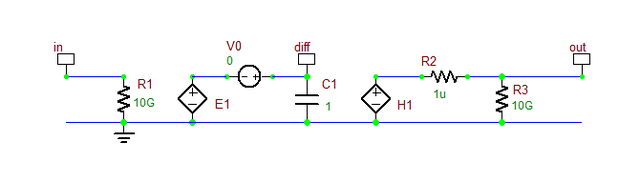An ideal differentiator using a capacitor.

The above figure shows a voltage differentiator macromodel using a 1F capacitor:

$E_1 = (1) v_{in} \\ i_C = \frac{dE_1}{dt} = \frac{dv_{in}}{dt} \\ H_1 = (1) i_C \\ v_{out} \approx H_1 = \frac{dv_{in}}{dt}$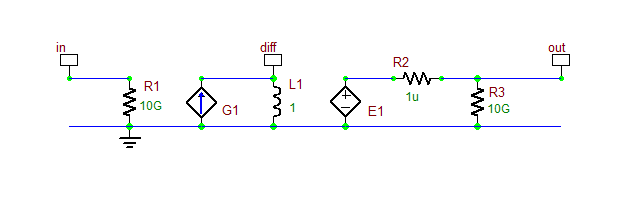An ideal differentiator using an inductor.

The above figure shows a voltage differentiator macromodel using a 1H inductor:

$G_1 = (1) v_{in} \\ v_L = v_{diff} = \frac{di_L}{dt} = \frac{dG_1}{dt} = \frac{dv_{in}}{dt} \\ E_1 = (1) v_{diff} \\ v_{out} \approx E_1 = \frac{dv_{in}}{dt}$

## An Analog Differentiator Macromodel

A more practical voltage differentiator circuit using an ideal operational amplifier (Op Amp) is shown in the figure below. The voltage transfer function of this circuit is given by:

$\frac{ v_{out} }{ v_{in} } = - \frac{R_1}{1/sC_1} = -s \left( R_1C_1 \right)$

which represents a derivative operator in the Laplace s-domain.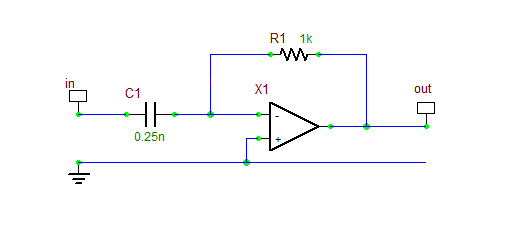A practical voltage differentiator circuit using an Op Amp.

An equivalent macromodel of the above circuit is given in the following figure. This is RF.Spice's "Analog Differentiator Block". In this model, the ideal Op Amp has been replaced with the linear controlled voltage source E2:

$E_2 = (-10^9) . v_{R_5}$

representing an open-loop gain of 1G. The nonlinear controlled source B1 is defined as:

$B_1 = -A . E_2 + v_{offset}$

where A is a gain parameter with a default unit value and voffset is an arbitrary offset voltage with a default zero value. The relationship between the input and output voltages is given by"

$v_{out}(t) \approx A . \frac{d}{dt} v_{in}(t) + v_{offset}$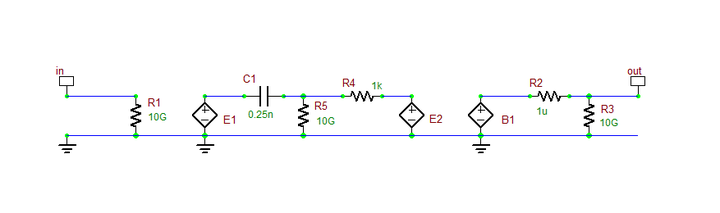RF.Spice's "Analog Differentiator" block circuit.

A practical voltage integrator circuit using an ideal Op Amp is shown in the opposite figure. The voltage transfer function of this circuit is given by:

$\frac{ v_{out} }{ v_{in} } = - \frac{1/sC_1}{R_1} = -\frac{1}{ s\left( R_1C_1 \right)}$

which represents a integral operator in the Laplace s-domain. This circuit is very similar to the Op Amp differentiator except for the fact that the timing resistor and capacitor have switched places.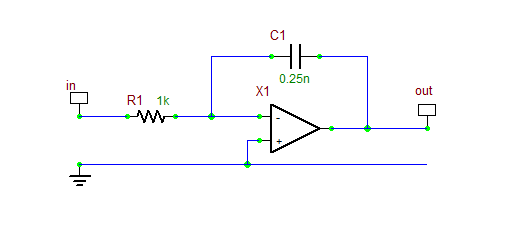A practical voltage integrator circuit using an Op Amp.

An equivalent macromodel of the above circuit is given in the following figure, which represents RF.Spice's "Analog Integrator Block". In this model, the ideal Op Amp has been replaced with the linear controlled voltage source E2:

$E_2 = (-10^9) . v_{R_6}$

representing an open-loop gain of 1G. The large shunt resistor R5 has been added to provide better DC stability. The nonlinear controlled source B1 is defined as:

$B_1 = A . E_2 + v_{offset}$

where A is a gain parameter with a default unit value and voffset is an arbitrary offset voltage with a default zero value.

The relationship between the input and output voltages is given by:

$v_{out}(t) \approx A . \int v_{in}(t) dt + v_{offset}$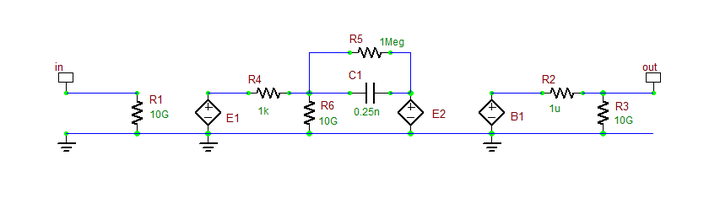RF.Spice's "Analog Integrator" block circuit.

## The Generalized Analog Filter Block

A general analog filter can be represented by a rational transfer function in the Laplace domain s-variable:

$H(s) = \frac{N(s)}{D(s)} = \frac{ \sum_{m=0}^{M} b_m s^m }{ \sum_{n=0}^{N} a_n s^n }$

In the above equation, we assume that M ≤ N and aN = 1. We normalize the numerator and denominator to the largest power of s:

$\frac{v_{out}}{v_{in}} = \frac{ \sum_{m=0}^{M} b_m s^m }{ s^N + \sum_{n=0}^{N-1} a_n s^n } = \frac{ \sum_{m=0}^{M} b_m s^{-(N-m)} }{ 1 + \sum_{n=0}^{N-1} a_n s^{-(N-n)} }$

In the above equation, the transfer function s-1 can indeed be realized using the analog integrator macromodel that was discussed earlier. Using a cascade of integrators one can realize the general rational transfer function as shown in the figure below: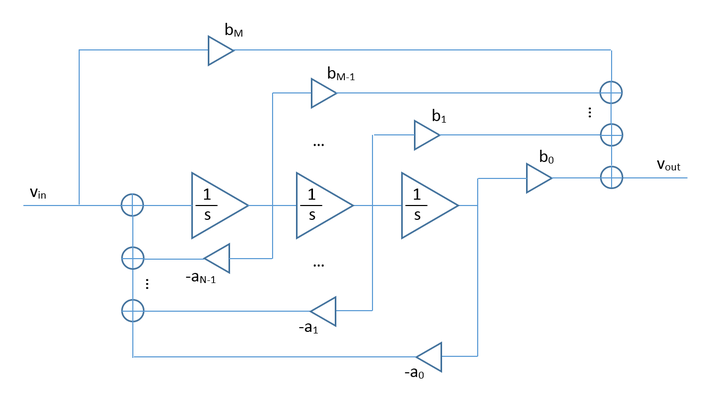The block diagram of a generalized analog filter with a rational s-domain transfer function.

RF.Spice's "Generalized Analog Filter Block" does this realization automatically for any arbitrary filter order (or highest degree of the s-variable). You enter the denominator and numerator coefficients as arrays of real numbers. The figure below shows the property dialog of this black-box macromodel.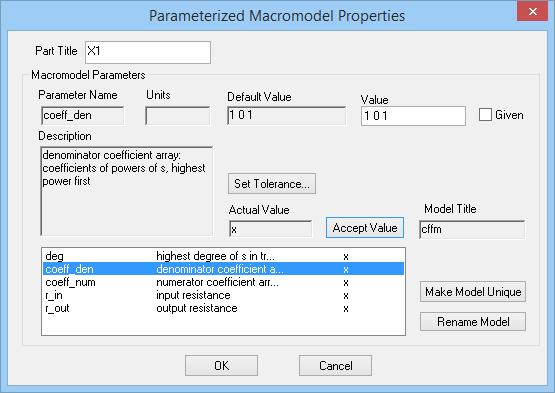The property dialog of the generalized analog filter block.

Note that XSpice provides a similar virtual block called "s-Domain Transfer Function Block" that also features a "Denormalization Angular Frequency" parameter expressed in rad/s. It also allows you to define gain and input offset parameters and set the initial condition for the integrators.

## A Digital Integrator Macromodel

The counterpart of an analog integrator with the transfer function s-1 in the digital signal processing world is a digital integrator with a Z-transform transfer function z-1. This is indeed a discrete-time delay operator. We can use the standard Spice model of a lossless transmission line (TRA) to introduce an arbitrary delay. A lossless transmission line model is characterized by its time delay TD and characteristic impedance Z0. The following figure shows the circuit of RF.Spice's "Digital Integrator Block". The transmission line model has Z0 = 50Ω and has been terminated in a 50Ω load. The time delay TD is set equal to half the sampling period (T/2). The linear controlled voltage source E3 has a gain of -1012 and acts as an ideal Op Amp voltage follower.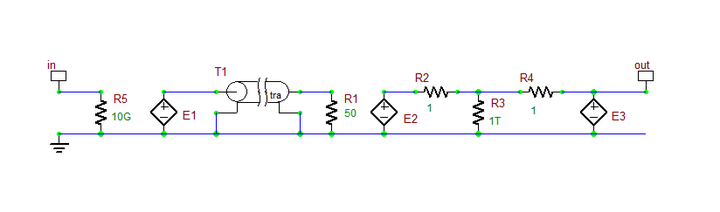RF.Spice's "Digital Integrator" block circuit.

The z-domain transfer function of the above digital integrator, called the DINT model in RF.Spice A/D, is given by:

$\frac{v_{out}(z)}{v_{in}(z)} = -z^{-1/2}$

Therefore, a cascade of two DINT devices is needed to obtain the transfer function z-1 corresponding to a time delay of T.

## The Generalized Digital Filter Block

Digital filters are generally classified into two groups: finite impulse response (FIR) filters and infinite impulse response (IIR) filters. FIR filter are built from forward delay elements, while IIR filters require negative feedback.

A general FIR filter can be defined by the simple following discrete convolution:

$y[n] = \sum_{k=0}^{N} h[k] . x[n-k]$

where h[n] is the impulse function of the filter and x[n] and y[n] are the input and output sequences, respectively. Note that h[n] has nonzero values only for 0 ≤ n ≤ N. x[n] can be regarded as the samples of a continuous-time signal x(t), which is samples at t = 0, T, 2T, ..., NT, where T is the sampling period.

The above discrete convolution equation can be transformed into the z-domain as follows:

$\frac{Y(z)}{X(z)} = H(z) = \sum_{k=0}^{N} h[k] z^{-k}$

It is clear that above FIR transfer function can be easily realized using a cascade of DINT devices with a nonlinear controlled (B-type) voltage source that gives the right weights to the delayed samples and sums them up at the output.

An IIR filter, on the other hand, has a z-domain transfer function of the following form:

A general analog filter can be represented by a rational transfer function in the Laplace domain s-variable:

$H(z) = \frac{N(z)}{D(z)} = \frac{ \sum_{m=0}^{M} b_m z^m }{ \sum_{n=0}^{N} a_n z^n }$

In the above equation, we assume that M ≤ N and aN = 1. This is the same as the transfer function of the generalized analog filter, where the Laplace s-variable has been replaced with he z-variable. Similarly, we normalize the numerator and denominator to the largest power of z:

$\frac{v_{out}}{v_{in}} = \frac{ \sum_{m=0}^{M} b_m z^m }{ z^N + \sum_{n=0}^{N-1} a_n z^n } = \frac{ \sum_{m=0}^{M} b_m z^{-(N-m)} }{ 1 + \sum_{n=0}^{N-1} a_n z^{-(N-n)} }$

In the above equation, the transfer function z-1 can be realized using two cascaded DINT macromodels as we discussed earlier. Using the cascade of digital integrators one can realize the general rational transfer function as shown in the figure below: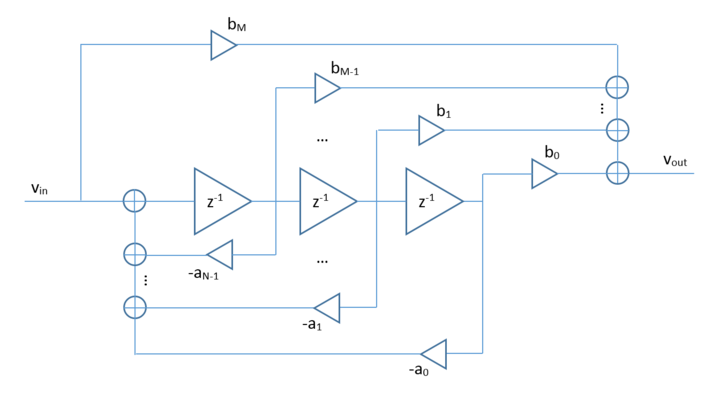The block diagram of a generalized IIR digital filter with a rational z-domain transfer function.

RF.Spice's "Generalized Digital Filter Block" does this realization automatically for any arbitrary filter order (or highest degree of the z-variable). Similar to the analog filter case, you enter the denominator and numerator coefficients as arrays of real numbers. But unlike the analog case, where the coefficients correspond to the powers of the s-variable, in the digital case the, you have to enter the coefficients for powers of (-z1/2). In other words, if the orders of the numerator and denominator polynomials are M and N, respectively, then you need to define 2M+1 coefficients for the numerator and 2N+1 coefficients for the denominator. Obviously, the odd-indexed coefficients would be all zeros in a typical rational transfer function. But they don't have to be so. The figure below shows the property dialog of this black-box macromodel.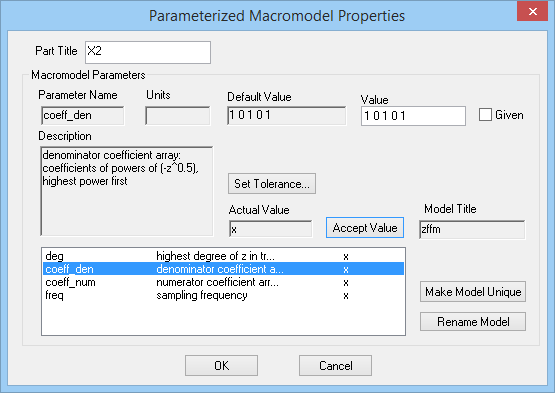The property dialog of the generalized digital filter block.

It can easily be seen that the FIR filter is a special case of the IIR z-domain transfer function when M = N and an = 0 for n = 0, 1, 2,..., N-1. In that case, one can write

$H(z) = \frac{1}{z^N} \sum_{m=0}^{M} b_m z^m = \sum_{m=0}^{M} b_m z^{-(N-m)} = \sum_{k=0}^{N} h[k] z^{-k}$

which implies that h[k] = bN-k. In other words, if you have the impulse response coefficients h[n] of a FIR filter, you enter them in the reverse order: h, h, ..., h[N-1], h[N], for the numerator array, and enter 1, 0, 0, ..., 0 for the denominator array. The figure below shows the reduced block diagram of the FIR digital filter.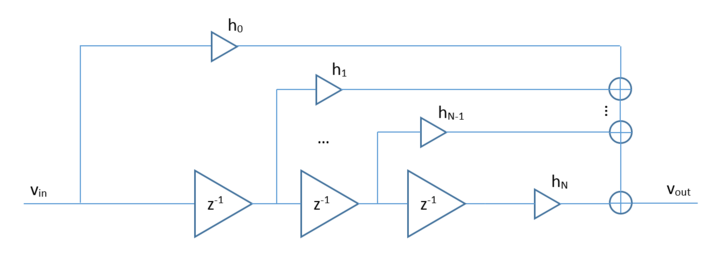The block diagram of a generalized FIR digital filter.

## Variety of Black-Box Virtual Blocks

RF.Spice A/D provides a large collection of black-box virtual blocks for different types of system applications. These have been loosely grouped into the following categories:

• Waveform Generation Blocks: including sinusoidal, triangular and square wave chirp generators, random noise generator, random pulse generator, etc.
• Analog Signal Processing Blocks: including signal conditioning and transformation blocks, two-signal operations and signal feature detection blocks.
• Digital Signal Processing Blocks: including sampling blocks, discrete-time Fourier transform (DFT) and discrete convolution blocks
• Spectral Processing Blocks: including voltage controlled oscillators (VCO), frequency conversion blocks, generic filters, phase locked loop (PLL), etc.
• Modulation Blocks: including analog and digital modulations schemes (FM, QAM, FSK, DPSK, Delta-Sigma, etc.)

You can access all these blocks from the Parts Menu. Some of the waveform generator devices are categorized as "voltage source" devices in the Device Manager's point of view. A number of the virtual blocks are native XSPICE models. You can find their models as "process models" in the Device Manager. Many others are "subscircuit models". You can open up, view and even modify their Netlist codes. Some other blocks like the generalized analog and digital filters do not have editable Netlist codes.

## A Note on the SPICE Simulation of Virtual Blocks

Most of the virtual blocks can be used in both transient and AC analysis tests. Obviously, nonlinear operations must be performed in the time domain. For example, you can see the performance of voltage controlled oscillators (VCO) only in a transient test, and using them in conjunction with AC frequency sweep analysis is meaningless. The same is true for frequency conversion blocks such as frequency multipliers. In addition, certain blocks such frequency conversion blocks may require harmonic wave input signals and may not work properly with arbitrary waveforms.

When your circuit involves several interconnected virtual blocks, the SPICE simulation of your circuit is likely to encounter convergence problems. This is more frequent when you a transient analysis of such circuits. In those case, we recommend that you change the SPICE integration method. You can do this by opening the SPICE Simulation Options dialog from the Simulate Menu. There are two options available: trapezoidal or gear. The default option is "trap". Changing the integration method to "gear" can improve the convergence of the transient analysis.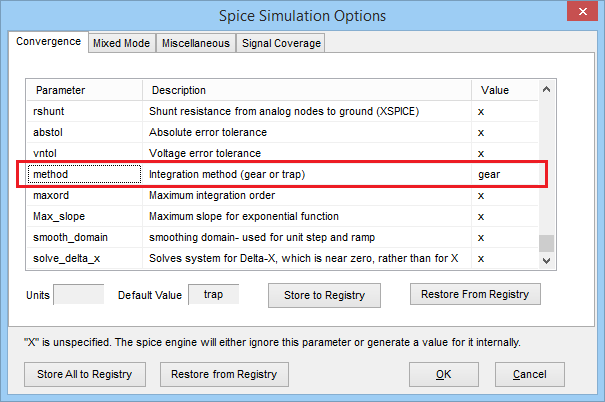Setting the integration method in the SPICE Simulation Options dialog.